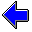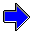Java Security Settings:
This web page employs Java, which requires specific security settings for correct operation.
If the applets on this page do not run correctly, consult the Virtual Chemistry Experiments FAQ
or the Physlet Physics web site for establishing the correct security settings.

# Crystal Field Theory

## Energy Level Splitting

The Crystal Field Theory experiment illustrates the effects on a metal d orbital energies of moving a set of negative point charges close to a metal ion. As one would expect, the energies of the d orbitals rise as the negative charges approach the metal ion, owing to the repulsions between the d orbital electrons and the surrounding charge.

If the surrounding negative charge is spherically symmetric, all five d orbitals are equally affected. In practice, the surrounding negative charge is never spherically distributed, because the charge is associated with specific ions that occupy specific positions. The consequence is each d orbital is affected differently, and how a particular d orbital is affected depends upon the geometry of the surrounding point charges. This effect is clearly seen in the splitting of the energy levels for the five d orbitals. When point charge enters a region of high electron density, the orbital energy rises significantly owing to the repulsion between the electron and the point charge. When the point charge approaches the ion along a nodal surface, the orbital energy does not increase as greatly.

The results from the Crystal Field Theory experiment are summarized in the chart shown below. Each geometry of point charges (linear, square planar, tetrahedral, or octahedral) produces a characteristic splitting pattern for the five d orbitals (xy, xz, yz, x2-y2, and z2). If you do not understand why the d orbitals split to form these specific patterns, revisit the previous experiment and carefully examine whether the point charges enter regions of high electron density or approach along nodal surfaces for a particular geometry and d orbital.The extent of the splitting in energies is represented by the splitting energy Δ. A subscript is typically employed to indicate the geometry of the surrounding point charges (t for tetrahedral and o for octahedral). The splitting is significantly smaller for a tetrahedral geometry than for an octahedral geometry: Δt = 4/9 Δo. (Why is Δt so much smaller than Δo? If necessary, revisit the Crystal Field Theory exercise and carefully compare the nature of the overlap of point charges and orbitals for the tetrahedral and octahedral cases.)

## Spectroscopy

 Let's return to our original question: Why is a ruby red? Recall that a ruby is the mineral corundum (Al2O3) containing trace amounts of Cr3+ ion in place of Al3+ ion in the corundum lattice. The Cr3+ ions are responsible for the color of a ruby. Each Al3+ ion (and thus each Cr3+ ion) in the corundum crystal is surrounded by six oxide ions in a distorted octahedral environment, as shown at right (the O2- ions are red and the Al3+ is blue). The electron configuration for Cr3+ is 1s22s22p63s23p63d3. (Recall that ionization of an atom removes an electron from the orbital with highest principle quantum.) The Cr3+ ion is thus a d3 ion. How are the three electrons distributed among the five 3d orbitals? As a slight simplification, let's assume the Cr3+ exists in an perfect octahedral environment. The 3d orbitals are thus split into two groups: xy, xz, and yz at lower energy and x2-y2 and z2 at higher energy. Recall that the lowest energy configuration is to place each electron in an orbital by itself with spin up (spin quantum number = +1/2). The electron configuration for Cr3+ in an octahedral environment is shown below at the left (ground-state electron configuration).Cr3+ ground-state electron configurationin octahedral environment The absorbance of light in the visible and ultraviolet regions of the spectrum is associated with promotion of an electron from a low-lying to a high-lying orbital. If a photon having an energy equal to Δo strikes Cr3+, the photon can be absorbed and an electron promoted from the low-lying set of three orbitals (designated t2g) to the high-lying set of two orbitals (designated eg). The electron configuration of the excited state is shown at the right. (Note that the xy, xz, and yz orbitals are equivalent, thus the electron may be removed from any of these orbitals. The x2-y2 and z2 orbitals are also equivalent, thus the promoted electron may be placed in either of these orbitals.)Cr3+ excited-state electron configurationin octahedral environment

 The absorbance of light may be measured using a spectrophotometer and displayed as an absorbance spectrum. The absorbance spectra of a ruby and an emerald are shown at the right. The lowest energy peaks arises from the t2g → eg transition described above, and thus the energy of that transition is Δo. photon energy = E = h ν = Δo In this equation, h = 6.62608 x 10-34 J sec is Planck's constant. The product of the frequency of the light, ν, and the wavelength of the light, λ, equals the speed of light, c = 2.997925 x 108 m/sec. For this particular ruby (there is significant variation in color between specimens), the lowest energy transition is centered at λ = 561 nm. The splitting energy is thus Δo = h c/λ = 3.54 x 10-19 J = 213 kJ/mol = 2.21 eVSpectra of a ruby from Chanthaburi, Thailand (red)and an emerald from Malyshevo, Ural, Russia (green). Data obtained from the Caltech Mineral Spectroscopy Server.

Note that various units that may be used to express this energy. Another common way to represent the energy is as the wave number, which is simply the reciprocal of the wavelength and has units of cm-1. You will observe that the energy is directly proportional to λ-1. The wave number for this transition is 17800 cm-1.

The corresponding transition for the emerald whose spectrum is shown at the right is 606 nm. The color of an emerald also arises from Cr3+ ions, in this case the Cr3+ ions are substituted for Al3+ ions in the beryl crystal structure. The Al3+ in both beryl and corundum are surrounded by oxide ions in an octahedral environment. Calculate Δo for the emerald using λ = 606 nm.

In most compounds, there are large gaps in energies between the bonding orbitals (which are typically filled with electrons) and the antibonding orbitals (which are typically empty). Promotion of an electron from an occupied bonding orbital to an unoccupied antibonding orbital (a σ → σ* transition) usually requires 6 eV or more energy. Such light falls in the ultraviolet region, which the human eye cannot detect, and the substance is colorless. Nonbonding orbitals (which are usually occupied) lie between the bonding and antibonding orbitals and thus give rise to lower energy transitions. These n → σ* transitions are usually 3.5 eV or greater, which still corresponds which light in the ultraviolet region (near ultraviolet light).

In order to have color, a substance must have an electronic transition whose energy change is between 1.8 and 3.1 eV, which corresponds with light in the visible range (about 400 to 700 nm). Such low energy transitions require closely spaced energy levels, some of which contain electrons and some of which do not. Many dyes are large organic molecules with extended π bonding, which gives rise to closely spaced occupied π and unoccupied π* orbitals. Transition metal complexes are often colored, because the crystal field splitting gives rise to closely spaced, partially filled d orbitals.

So why does the absorbance spectrum for ruby result in a red color while that for emerald results in a green color?Crystal Field Theory                     Color in GemsCoordination Chemistry Home PageVirtual Chemistry Experiments Home Page

Splitting.html version 1.1